Examples

Chapter 6 Class 12 Application of Derivatives
Serial order wise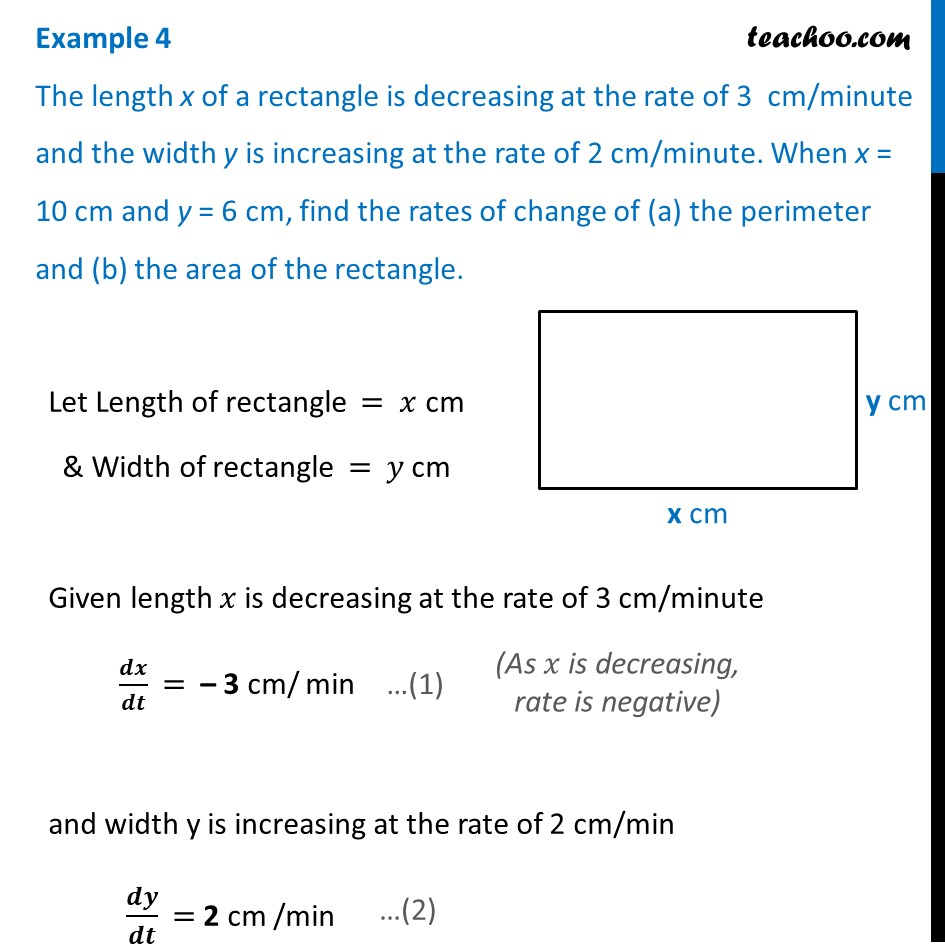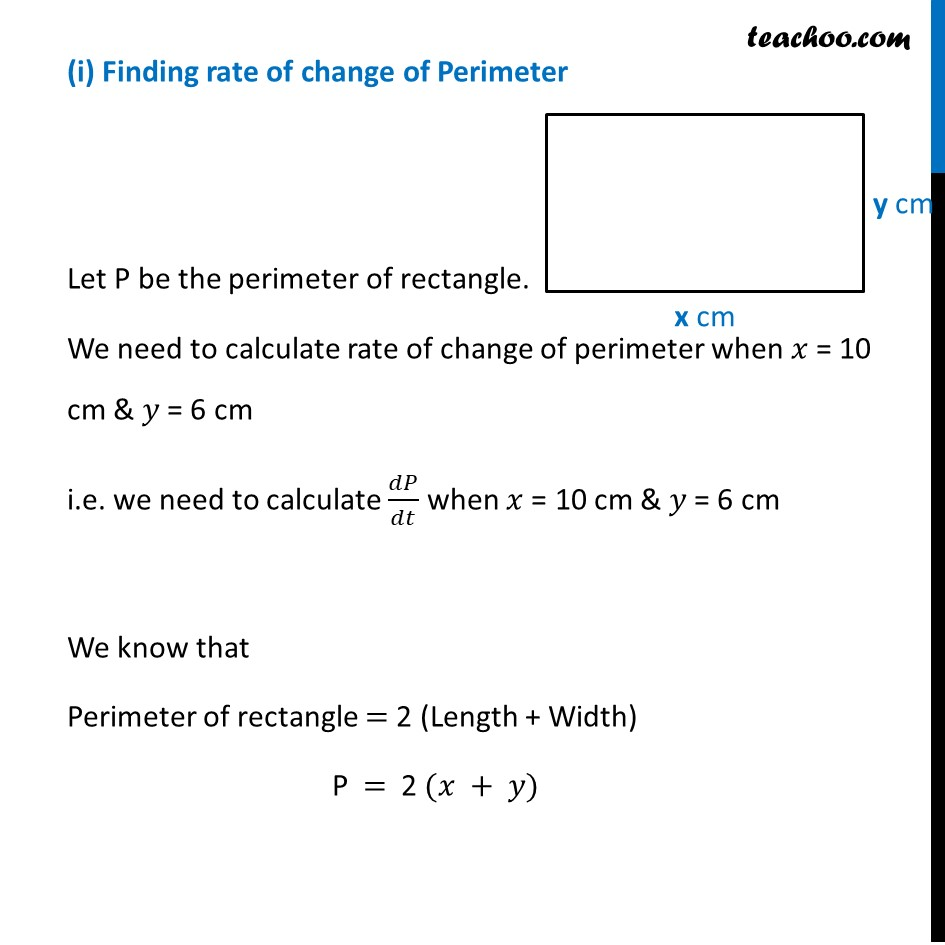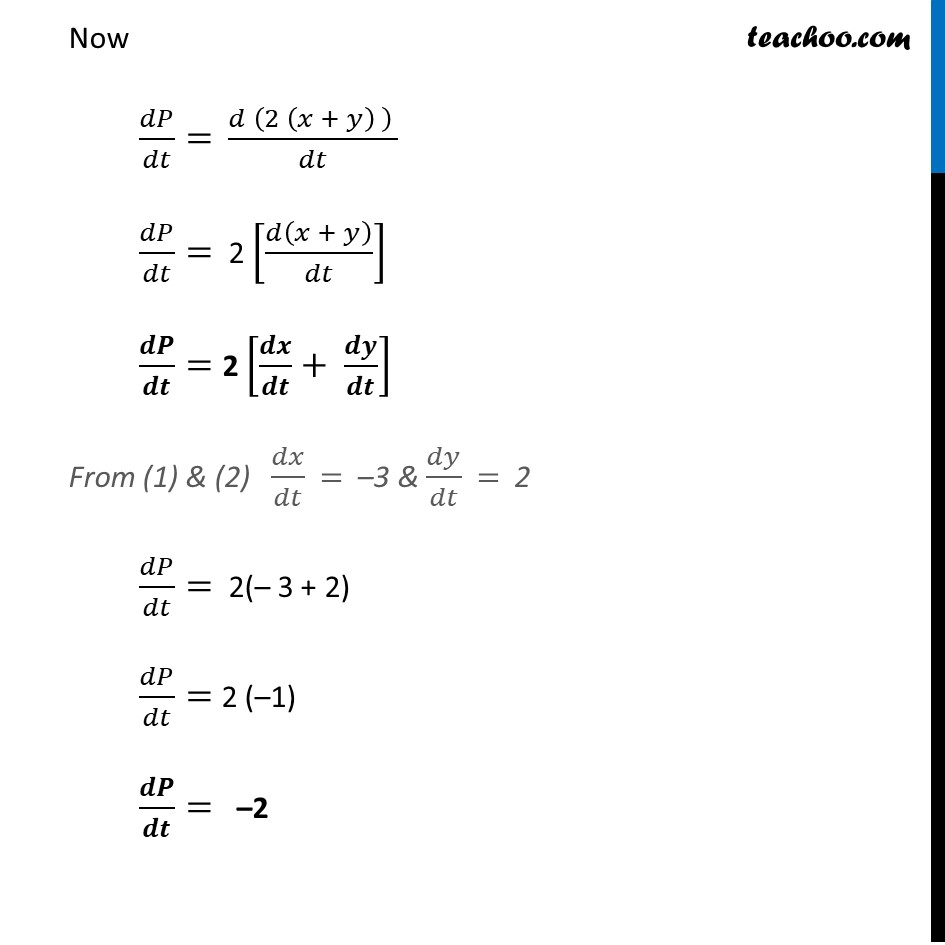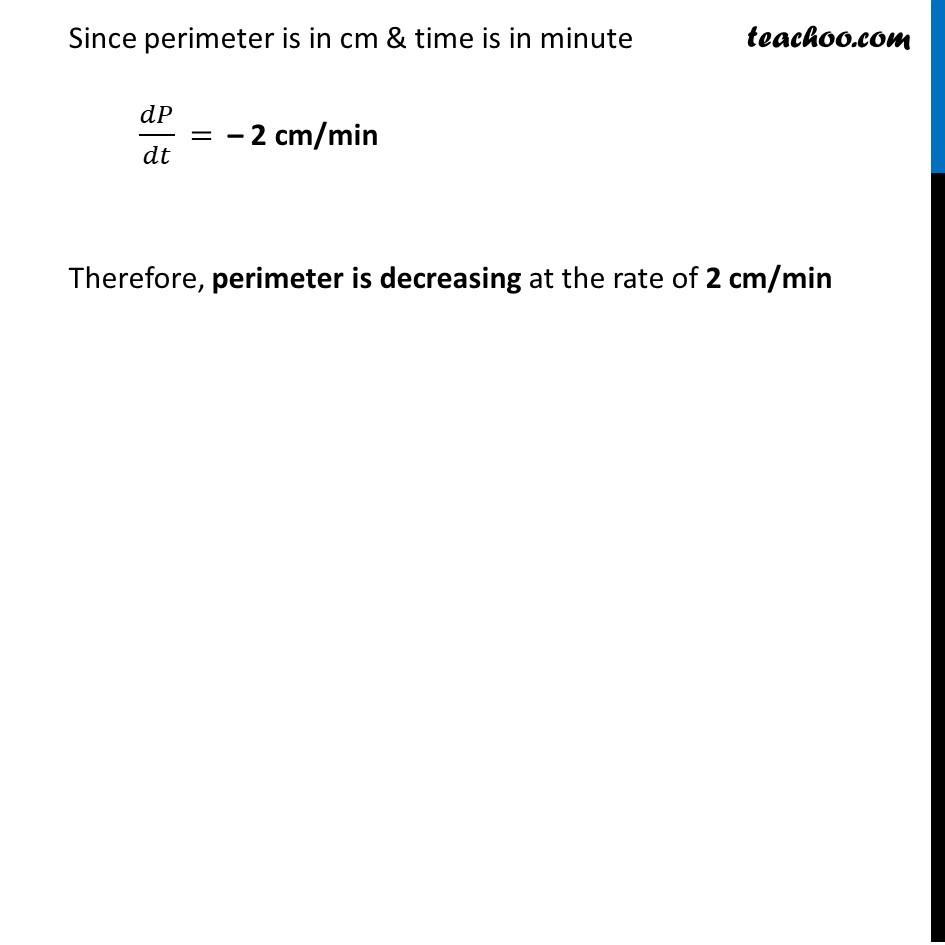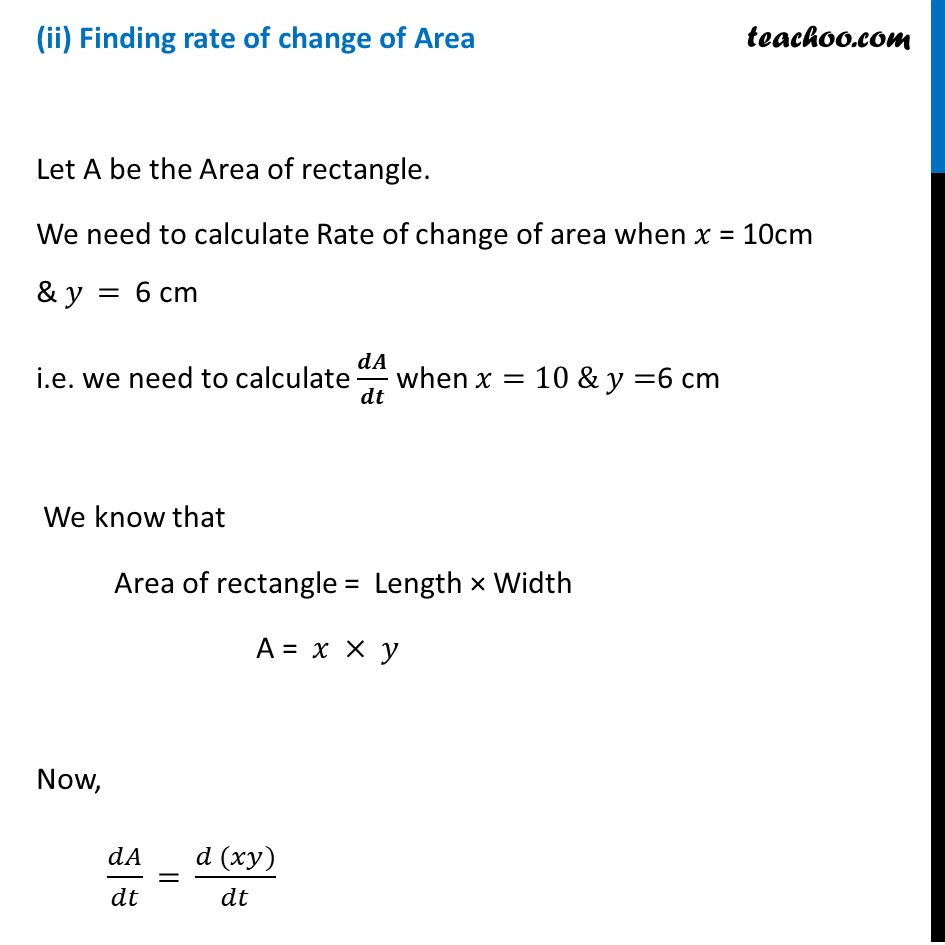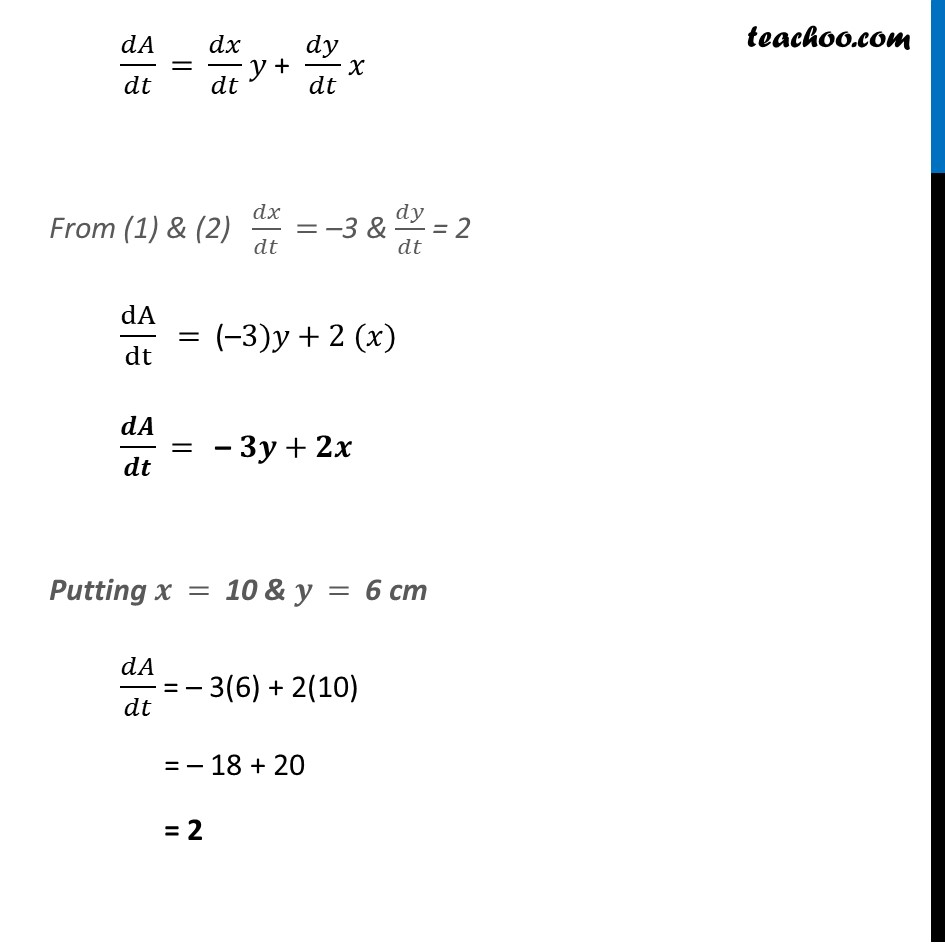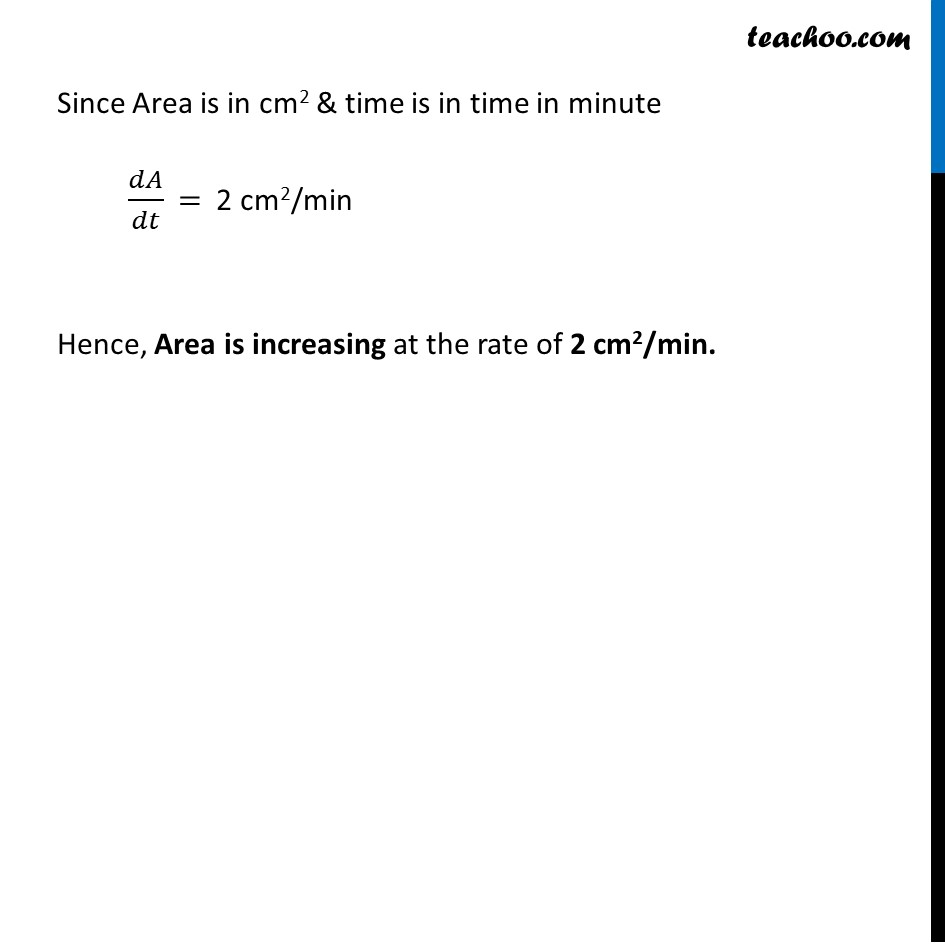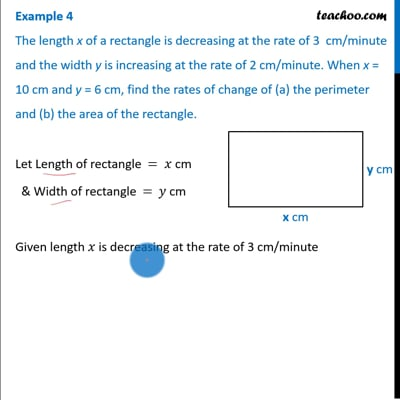This video is only available for Teachoo black users

Maths Crash Course - Live lectures + all videos + Real time Doubt solving!

### Transcript

Example 4 The length x of a rectangle is decreasing at the rate of 3 cm/minute and the width y is increasing at the rate of 2 cm/minute. When x = 10 cm and y = 6 cm, find the rates of change of (a) the perimeter and (b) the area of the rectangle.Let Length of rectangle = 𝑥 cm & Width of rectangle = 𝑦 cm Given length 𝑥 is decreasing at the rate of 3 cm/minute 𝒅𝒙/𝒅𝒕 = – 3 cm/ min and width y is increasing at the rate of 2 cm/min 𝒅𝒚/𝒅𝒕 = 2 cm /min (i) Finding rate of change of Perimeter Let P be the perimeter of rectangle. We need to calculate rate of change of perimeter when 𝑥 = 10 cm & 𝑦 = 6 cm i.e. we need to calculate 𝑑𝑃/𝑑𝑡 when 𝑥 = 10 cm & 𝑦 = 6 cm We know that Perimeter of rectangle = 2 (Length + Width) P = 2 (𝑥 + 𝑦) Now 𝑑𝑃/𝑑𝑡= (𝑑 (2 (𝑥 + 𝑦) ) )/𝑑𝑡 𝑑𝑃/𝑑𝑡= 2 [𝑑(𝑥 + 𝑦)/𝑑𝑡] 𝒅𝑷/𝒅𝒕= 2 [𝒅𝒙/𝒅𝒕+ 𝒅𝒚/𝒅𝒕] From (1) & (2) 𝑑𝑥/𝑑𝑡 = –3 & 𝑑𝑦/𝑑𝑡 = 2 𝑑𝑃/𝑑𝑡= 2(– 3 + 2) 𝑑𝑃/𝑑𝑡= 2 (–1) 𝒅𝑷/𝒅𝒕= –2 Since perimeter is in cm & time is in minute 𝑑𝑃/𝑑𝑡 = – 2 cm/min Therefore, perimeter is decreasing at the rate of 2 cm/min(ii) Finding rate of change of Area Let A be the Area of rectangle. We need to calculate Rate of change of area when 𝑥 = 10cm & 𝑦 = 6 cm i.e. we need to calculate 𝒅𝑨/𝒅𝒕 when 𝑥=10 & 𝑦=6 cm We know that Area of rectangle = Length × Width A = 𝑥 × 𝑦 Now, 𝑑𝐴/𝑑𝑡 = (𝑑 (𝑥𝑦))/𝑑𝑡 𝑑𝐴/𝑑𝑡 = 𝑑𝑥/𝑑𝑡 𝑦 + 𝑑𝑦/𝑑𝑡 𝑥 From (1) & (2) 𝑑𝑥/𝑑𝑡 = –3 & 𝑑𝑦/𝑑𝑡 = 2 dA/dt = (–3)𝑦+2 (𝑥) 𝒅𝑨/𝒅𝒕 = – 𝟑𝒚+𝟐𝒙 Putting 𝒙 = 10 & 𝒚 = 6 cm 𝑑𝐴/𝑑𝑡 = – 3(6) + 2(10) = – 18 + 20 = 2 Since Area is in cm2 & time is in time in minute 𝑑𝐴/𝑑𝑡 = 2 cm2/min Hence, Area is increasing at the rate of 2 cm2/min.